Created March 25, 2017

# Cleaneous maximus

People need a new, exciting, and irresistable way to keep the environment clean!

232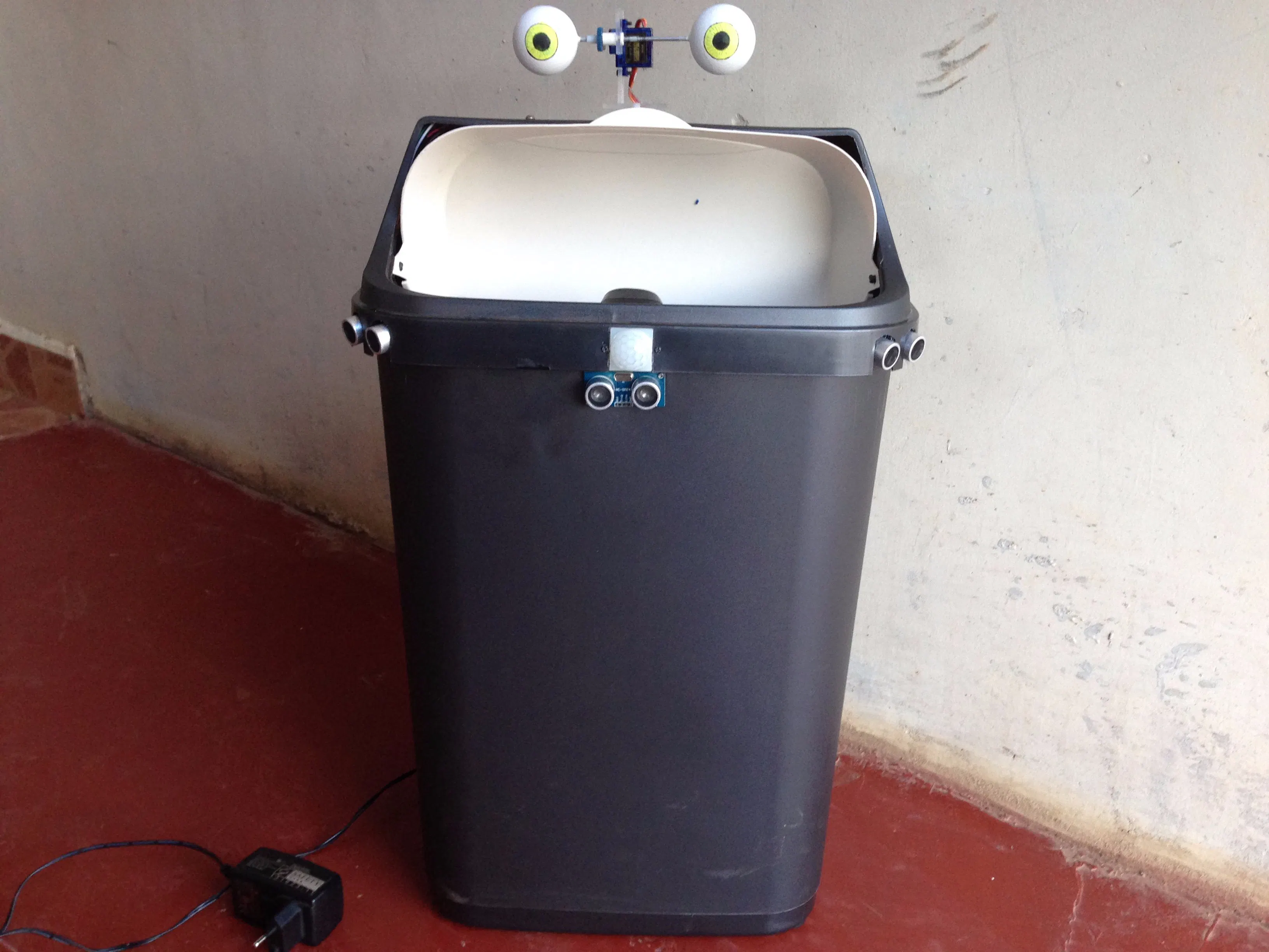## Things used in this project

### Hardware components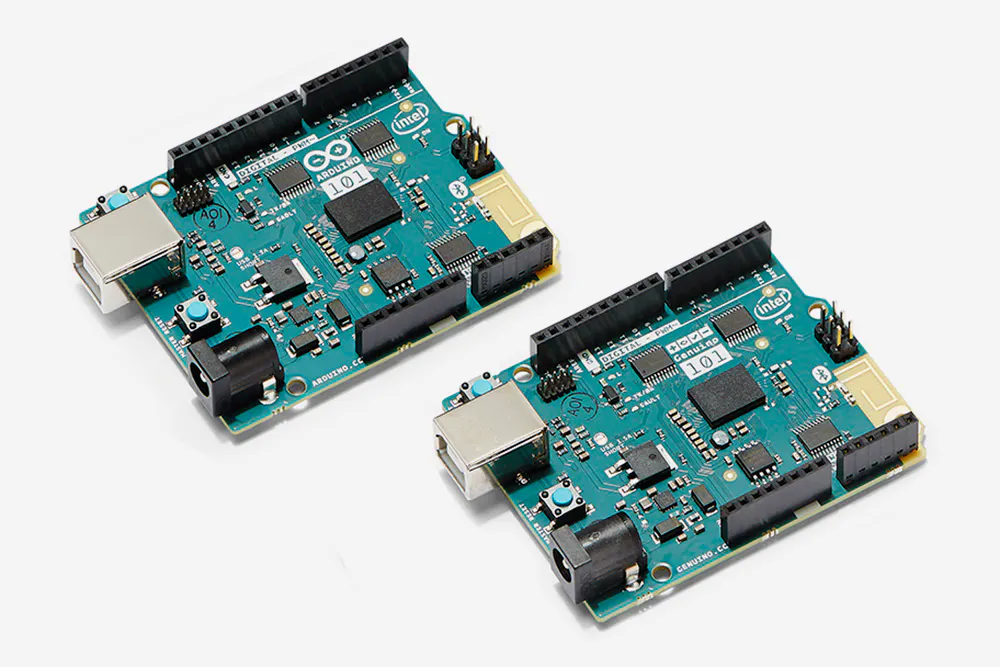Arduino 101 & Genuino 101
×1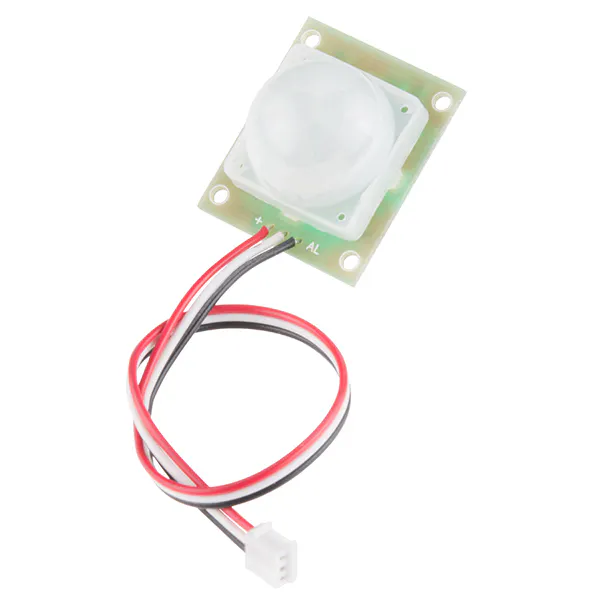PIR Motion Sensor (generic)
×2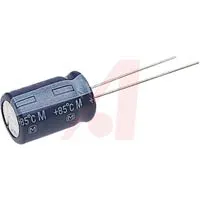Capacitor 10 µF Used with the external voltage regulator
×2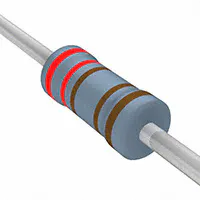Resistor 2.21k ohm One for each ultrasonic
×3Jumper wires (generic)
×1Ultrasonic Sensor - HC-SR04 (Generic)
×3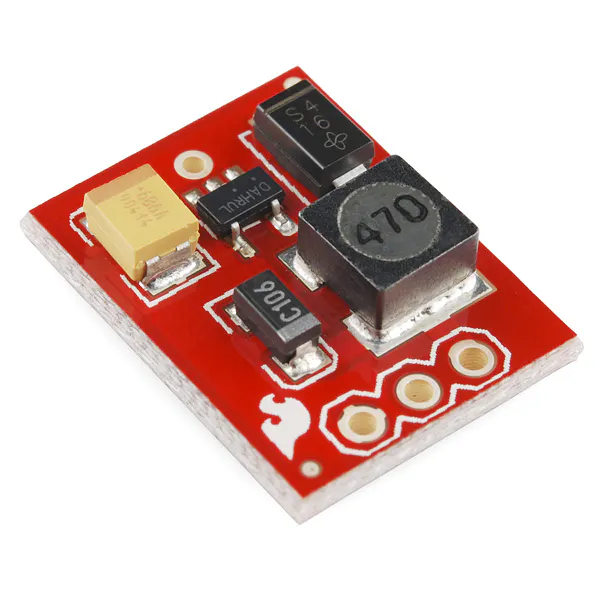SparkFun Step-Up Voltage Regulator - 5V
×1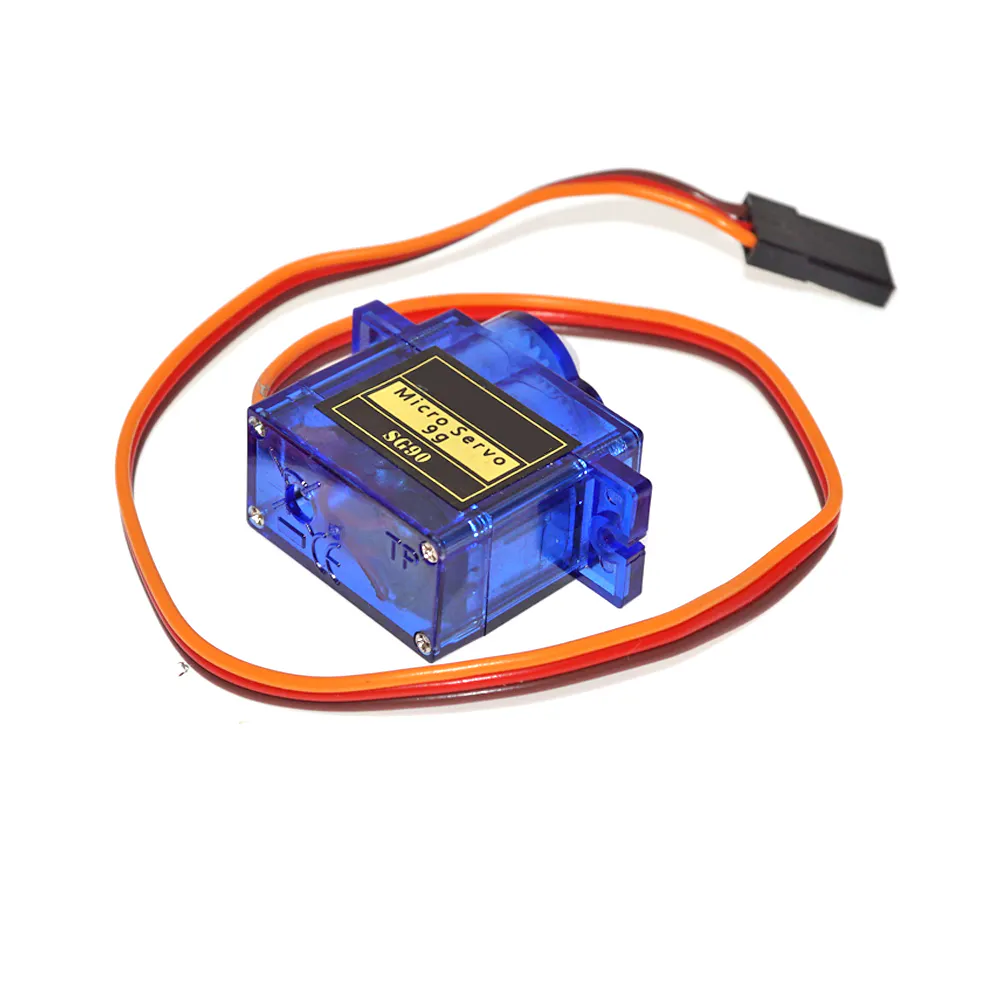SG90 Micro-servo motor
×2
 Micro SD card module for Arduino
×1
 TRS 3.5mm Audio Jack
×1
 3.5mm capable amplifier
×1

### Software apps and online servicesMicrosoft Visual Studio 2015Arduino IDE
 Github

### Hand tools and fabrication machinesHot glue gun (generic)Soldering iron (generic)
 Super glue
 drill-bits
 Grit paper

## Custom parts and enclosures

### Eye structure

Drawn using Autodesk Inventor 2016. This is the design we came up with for the eye structure that gives 2 sychronous eye movements.

## Schematics

### Project schematics

This is a circuit diagram that shows how the various components have been connected. Done in Fritzing.

## Code

### Cleaneous brain

C/C++
This is the code file that puts together all the sensors and actuators cleaneous uses.
```/*
Name:		Bincode.ino
Created:	3/10/2017 4:23:02 PM
Author:	Anthony_Brown
*/

#include <Servo.h>
#include <CurieTimerOne.h>
#include <SPI.h>
#include <SD.h>
#include <Arduino.h>
#include <aux_regs.h>
#include <conf.h>
#include <scss_registers.h> //This gives us the register addresses for the quark.
#include <stdio.h>
#include <math.h>

//AUDIO TYPES
#define passerby 1
#define approach 2
#define deposit 3
#define leaving 4

// AUDIO AND TIMER VARIABLES
uint32_t pwmChan;						//Will be set to quark timer 0 for the pwm DAC
const uint32_t clock_freq = 32000000;	//The frequncy of the Quark clock.(32Mhz)
const uint32_t pwm_frequency = 400000;	//The desired frequency of the pwm DAC
const int sampler_input_pin = 8;		//This pin will receive the sampling interrupt generated by Quark. Timer 4
const int sampler_output_pin = 9;		//This is th pin through which the timer will generate the sampling interrupt.
//sampler_input_pin and sampler_output_pin should therefore be shorted.

#define BUFFERSIZE 256					//This is the amount of data to be fetched from the sd card for each read.
const int speaker_pin = 3;				//Pin to which speaker will be connected

//This struct holds information related to the wav file that's being read.
typedef struct
{
int format;
int sample_rate;
int bits_per_sample;
}wave_format;
wave_format wave_info;

volatile unsigned char note = 0;		//holds the current voltage value to be sent to the ADC
volatile int period;
unsigned char buffer1[BUFFERSIZE], buffer2[BUFFERSIZE]; //Two cycling buffers which contain the wav data.
char file_name;						//wav file name.
unsigned long file_size;				//will store the size of the audio file in bytes.
char play_buffer = 0;					//keeps track of which buffer is currently being used
char new_buffer_ready = 0;				//Flag used by loop code to tell the interrupt that new data is ready in the buffer.
volatile unsigned int byte_count = 0;	//keeps track of the read position in the current buffer.
volatile char need_new_data = 0;		//Flag used by interrupt to tell 'Loop' code that a buffer is empty and needs to be refilled.
bool ramp_down = 0;
volatile int ramp_factor = 0;			// This will be increased progressively to alter playback volume at end of file.
File audio;

//SENSOR VARIABLES
volatile int pass_count = 0;
int pingright = 4;
int pingleft = 0;
int pingcenter = 1;
int PIRpin = 2;
int throwPIR = 7;
int levelping = 0;
unsigned long lastapproach = 0;
unsigned long lastthrow = 0;
unsigned long lastpass = 0;
volatile bool throwin = 0;
volatile bool motion = 0; //this flag will be raised when motion is detected after stillness.
volatile bool approached = 0;

struct proxi_multi
{
int distance;
int sensor;
};

//EYE VARIABLES
const int pan = 6;
const int tilt = 5;
int deftilt = 90;
int defpan = 90;
Servo panservo;
Servo tiltservo;
const int maxpan = 150;
const int minpan = 30;
const int maxtilt = 120;
const int tiltdef = 60;
bool eyereset = 0;

//ENVIRONMENT CONSTANTS
#define avgheight 150
#define binheight 130

//this function selects an audiofile from the passed category to be played at random
const char* audio_select(int audiotype)
{
Serial.print("selecting audio...\t");
int filecount = 1;
while (filecount < 99)
{
char name;
sprintf(name, "%d%.2d.wav", audiotype, filecount);
if (SD.exists(name))
filecount++;
else
break;
}
Serial.print(filecount);
Serial.print(" files of type ");
Serial.print(audiotype);
static char filename;
randomSeed(millis());
int audionum = random(1, filecount);
sprintf(filename, "%d%.2d.wav", audiotype, audionum);
const char *str = filename;
Serial.print("...selected audio = ");
Serial.println(str);
return str;
}

//Setup the constant parameters of a square wave on quark timer 0
void pwm_setup(int pin)
{
PinDescription *p = &g_APinDescription[pin];
//convert pin to channel:
if (p->ulPwmChan == INVALID)
{
Serial.println("Invalid pin Number for timer");
return; //The pin you gave is not pwm enabled.
}
pwmChan = p->ulPwmChan;
SET_PIN_PULLUP(p->ulSocPin, 0);
SET_PIN_MODE(p->ulSocPin, PWM_MUX_MODE);
p->ulPinMode = PWM_MUX_MODE;

//Configure for pwm mode, no interrupts, free running, enabled
offset = (pwmChan * QRK_PWM_N_REGS_LEN) + QRK_PWM_N_CONTROL;
QRK_PWM_CONTROL_PWM_OUT |		//sets to pwm mode
QRK_PWM_CONTROL_INT_MASK |		//disables interupts for now
QRK_PWM_CONTROL_MODE_PERIODIC |	//user defined count
QRK_PWM_CONTROL_ENABLE;			//enable timer
}

//this ISR is called to retrieve and play the next sample from the audio file.
void sampleISR()
{
noInterrupts();					//Disable interrupts
//Check to see if we've read all of the data in the current buffer
if (byte_count == BUFFERSIZE)
{
need_new_data = 1;			//Set a flag to tell the 'loop' code to refill the current buffer.
byte_count = 0;				//Reset the count
//Check to see if new data exists in the alternate buffer
{
//If new data is available, reassign the play buffer.
if (play_buffer == 0)
play_buffer = 1;
else
play_buffer = 0;
}
else
{
//If no new data is available then wait for it!
interrupts();
return;
}
}
//Find out which buffer is being used, and get data from it.
if (play_buffer == 0)
note = buffer1[byte_count];
else
note = buffer2[byte_count];
//Increase the byte_count since we've taken the current data.
byte_count++;
if (ramp_down)
{
ramp_factor += 10;
}
//Update the pwm with the retrieved value from the play buffer having subtracted the ramp_factor
if (note - ramp_factor < 0)
note = 0;			//we dont want a negative amplitude.
else
note = note - ramp_factor;
pwm_update(note);		//The note retrieved is passed as the duty cycle to the pwm timer.
interrupts();			//Re-enable interrupts
}

//This function updates the quark timer 0 with required duty
void pwm_update(int duty)
{
double ticks = clock_freq / pwm_frequency; //Number of 32mhz periods for one period of desired freq
uint32_t high_time = round(ticks * duty / 255.00);
uint32_t low_time = ticks - high_time;
offset = (pwmChan * QRK_PWM_N_REGS_LEN) + QRK_PWM_N_LOAD_COUNT1;
MMIO_REG_VAL(QRK_PWM_BASE_ADDR + offset) = low_time; //put low time into load count 1
offset = (pwmChan * QRK_PWM_N_REGS_LEN) + QRK_PWM_N_LOAD_COUNT2; //put high_time into load count 2
}

//sets up a timer that will generate an interrupt at the passed sample_rate(kH) throught the passed pin
void setup_timer(int sample_rate, int pin)
{
double interval = 1000000.00 / sample_rate;
uint32_t offset;
PinDescription *p = &g_APinDescription[pin];
uint32_t timerChan = p->ulPwmChan;

//Configure for timer mode, with interrupts, free running , enabled.
offset = (timerChan * QRK_PWM_N_REGS_LEN) + QRK_PWM_N_CONTROL;
QRK_PWM_CONTROL_MODE_PERIODIC |	//user defined count
QRK_PWM_CONTROL_ENABLE;			//enable timer

//set timer count. The timer will expire at this point and call the interrupt
uint32_t count = interval * clock_freq / 1000000;		//number of 32Mhz ticks to count
offset = (timerChan * QRK_PWM_N_REGS_LEN) + QRK_PWM_N_LOAD_COUNT1;
//connect timer to the pin through which it will send the signal
SET_PIN_PULLUP(p->ulSocPin, 0);
SET_PIN_MODE(p->ulSocPin, PWM_MUX_MODE);
p->ulPinMode = PWM_MUX_MODE;
//Attatch the SampleISR to the sampler input pin.
attachInterrupt(digitalPinToInterrupt(sampler_input_pin), sampleISR, CHANGE);
}

void disable_timer(int pin)
{
Serial.println("disable_timer");
uint32_t offset;
PinDescription *p = &g_APinDescription[pin];
uint32_t timerChan = p->ulPwmChan;
offset = (timerChan * QRK_PWM_N_REGS_LEN) + QRK_PWM_N_CONTROL;
QRK_PWM_CONTROL_DISABLE ;
}
/*
{
char field;
wave_info.sample_rate = (int)strtol(field, NULL, 16);
wave_info.format = (int)strtol(field, NULL, 16);
wave_info.bits_per_sample = (int)strtol(field, NULL, 16);
Serial.print("bits per sample = ");							//print the wav info
Serial.print(wave_info.bits_per_sample);
Serial.print("...sample_rate = ");
Serial.print(wave_info.sample_rate);
Serial.print("...format = ");
Serial.println(wave_info.format);
return;
}
*/

void playaudio(const char *filename)
{
audio = SD.open(filename);
//confirm if the file was found and check it's size.
if (audio)
{
file_size = audio.size();
Serial.print(filename);
Serial.print(" opened succesfully. File size = ");
Serial.println(file_size);
}
else {
Serial.println("No such file");
return;
}
pwm_setup(speaker_pin);			//start the pwm wave on a certain pin.
int bytes_read = 0;				//Keeps track of how many bytes are read when accessing a file on the SD card.
audio.seek(45);
unsigned long ramp_point = audio.size() - 256;
play_buffer = 0;												//set the initial play buffer, and grab the initial data from the SD card
setup_timer(16000, sampler_output_pin);			//start the sample rate timer which also attatches the sampler input interrupt.
while (1)
{
if (need_new_data == 1)		//need_new_data flag is set by ISR to indicate a buffer is empty and should be refilled
{
need_new_data = 0;		//clear the flag.
if (play_buffer == 0)	//play buffer indicates which buffer is now empty
{
bytes_read = audio.read(buffer1, BUFFERSIZE);		//Get the next BUFFERSIZE bytes from the file.
}
else
{
bytes_read = audio.read(buffer2, BUFFERSIZE);		//get the next BUFFERSIZE bytes from the file.
}
new_buffer_ready = 1;	//new_buffer_ready flag tells the ISR that the buffer has beed filled.
if (audio.position() >= ramp_point && !ramp_down)		//Check if we are near the end of the file and ramp down if we are.
{
ramp_down = 1;		//Raise the ramp_down flag.
}
//If file_read returns 0 or -1 file is over. Close the file, disable timers and exit.
{
//detachInterrupt(sampler_input_pin);
disable_timer(speaker_pin);
disable_timer(sampler_output_pin);
if (audio)
audio.close();
audio.rewindDirectory();
Serial.print("playback over:");
break;
}
}
}
ramp_factor = 0; //Reset the ramp_factor to 0 and drop the ramp_down flag for the next audio.
ramp_down = 0;
Serial.println("exiting player");
return;
}

//checks the proximity on a specifiec sensor.
long proximity(int pingPin)
{
unsigned long duration;
int min = 0;
for (int i = 1; i <= 3; i++)
{
pinMode(pingPin, OUTPUT);
digitalWrite(pingPin, LOW);
delayMicroseconds(2);
digitalWrite(pingPin, HIGH);
delayMicroseconds(5);
digitalWrite(pingPin, LOW);
pinMode(pingPin, INPUT);
duration = pulseIn(pingPin, HIGH);
long dist = duration / 29 / 2;
if (i == 1)
min = dist;
else if (dist != 0 && dist < min)
min = dist;
delay(100);
}
if (min == 0) {
return 400; //probably the ultrasounds are maxing out because there is nobody.
}
else {
return min;
}
}

//checks the proximity on all three sensors and returns the shortest distance of the three
struct proxi_multi proximity_multiple()
{
struct proxi_multi d;
int prox1 = proximity(pingright);
int prox2 = proximity(pingcenter);
int prox3 = proximity(pingleft);
int min = prox1;
d.sensor = pingright;
if (prox2 < min) {
min = prox2;
d.sensor = pingcenter;
}
if (prox3 < min) {
min = prox3;
d.sensor = pingleft;
}
d.distance = min;
return d;
}

//moves the eye to desired pan and tilt at passed speed which is time between angles.
void eye_move(int pan, int tilt, int speed = 0)
{
eyereset = 0;
int pandiff = abs(pan - lastpan);
int tiltdiff = abs(tilt - lasttilt);
float p, t;
if (tiltdiff >= pandiff)
{
float ratio = pandiff / tiltdiff;
if (tilt >= lasttilt)
{
if (pan >= lastpan)
{
for (t = lasttilt, p = lastpan; t < tilt; t++, p += ratio)
{
tiltservo.write(t);
panservo.write(round(p));
delay(speed);
}
}
else if (pan < lastpan)
{
for (t = lasttilt, p = lastpan; t < tilt; t++, p -= ratio)
{
tiltservo.write(t);
panservo.write(p);
delay(speed);
}
}
}
else if (tilt < lasttilt)
{
if (pan >= lastpan)
{
for (t = lasttilt, p = lastpan; t > tilt; t--, p += ratio)
{
tiltservo.write(t);
panservo.write(round(p));
delay(speed);
}
}
else if (pan < lastpan)
{
for (t = lasttilt, p = lastpan; t > tilt; t--, p -= ratio)
{
tiltservo.write(t);
panservo.write(round(p));
delay(speed);
}
}
}
}
else if (pandiff > tiltdiff)
{
float ratio = tiltdiff / pandiff;
if (pan >= lastpan)
{
if (tilt >= lasttilt)
{
for (p = lastpan, t = lasttilt; p < pan; p++, t += ratio)
{
panservo.write(p);
tiltservo.write(round(t));
delay(speed);
}
}
else if (tilt < lasttilt)
{
for (p = lastpan, t = lasttilt; p < pan; p++, t -= ratio)
{
panservo.write(p);
tiltservo.write(round(t));
delay(speed);
}
}
}
else if (pan < lastpan)
{
if (tilt > lasttilt)
{
for (p = lastpan, t = lasttilt; p > pan; p--, t += ratio)
{
panservo.write(p);
tiltservo.write(round(t));
delay(speed);
}
}
else if (tilt < lasttilt)
{
for (p = lastpan, t = lasttilt; p > pan; p--, t -= ratio)
{
panservo.write(p);
tiltservo.write(round(t));
delay(speed);
}
}
}
}
}

void looktomin (struct proxi_multi surround)
{
if (surround.sensor == pingleft)
{
//double tanratio = (avgheight-binheight) / surround.distance;	//get the tilt angle in degrees
//double angle = atan(tanratio) * deginrad;
eye_move(maxpan, 70, 10);					//look to the right
}
else if (surround.sensor == pingright)
{
//double tanratio = (avgheight-binheight) / surround.distance;	//get the tilt angle in degrees
//double angle = atan(tanratio) * deginrad;
eye_move(minpan, 70, 10);						//look to the left
}
else
{
//double tanratio = (avgheight - binheight) / surround.distance;	//get the tilt angle in degrees
//double angle = atan(tanratio) * deginrad;
eye_move(defpan, 70, 10);						//look straight ahead
}
}
//This in an ISR called every time the the outer PIR detects motion after a period of inactivity.
void motiondetect()
{
motion = 1;
}

//will be caled whenever the motion flag is up.
void motion_action()
{
unsigned long motiontime = millis();//stores the time when motion was detected
//detachInterrupt(digitalPinToInterrupt(PIRpin)); //detatch the outer pir interrupt to avoid it interrupting playbackwhi
proxi_multi minsurround = proximity_multiple();
if (!throwin)
{
while (millis() - motiontime < 2000 && minsurround.distance>50 && !approached)
{
struct proxi_multi surroundtest = proximity_multiple();
if (surroundtest.distance < minsurround.distance)
minsurround = surroundtest;
}
if (minsurround.distance <= 50) //it means the person has come close. to the dustbin
{
if (!approached)
{
looktomin(minsurround);
playaudio(audio_select(approach));
approached = 1; //raise the approached flag.
lastapproach = millis();
Serial.println("approached");
}
}
else if (minsurround.distance > 50 && millis() - lastpass > 5000)	//they dont seem to be approaching.
{
pass_count++;
lastpass = millis();
Serial.println("passer-by");
if (pass_count == 5)
{
playaudio(audio_select(passerby));
pass_count = 0;
}
}
}
//else
//{
// while (approached && minsurround.distance < 50 && millis() - lastapproach < 10000) //they are leaving
// {
//	 struct proxi_multi surroundtest = proximity_multiple();
//	 if (surroundtest.distance < minsurround.distance)
//		 minsurround = surroundtest;
// }
// if (minsurround.distance > 50)
// {
//	 playaudio(audio_select(leaving));
//	 motion = 0; //drop the motion flag
//	 approached = 0; //drop the aproached flag.
//	 throwin = 0;
// }
//
//}
}

//Called when throwin flag is up
void throw_action()
{
if (approached)
{
Serial.println("throwin");
playaudio(audio_select(deposit));
lastthrow = millis();
delay(1000);
playaudio(audio_select(leaving));
throwin = 0;
eye_move(defpan, deftilt, 10);
}
}
void throwdetect()
{
throwin = 1;
}

void setup() {
Serial.begin(115200);
if (!SD.begin(10))
{
Serial.println("Failed to initiallize SD");
return;
}
else
Serial.println("SD initialized succesfully");
pinMode(sampler_input_pin, INPUT);
//attatch an interrupt to the PIR that will trigger a response from the bin.
attachInterrupt(digitalPinToInterrupt(PIRpin), motiondetect, RISING);
attachInterrupt(digitalPinToInterrupt(throwPIR), throwdetect, RISING);
panservo.attach(pan);
tiltservo.attach(tilt);
panservo.write(90);
tiltservo.write(deftilt);
delay(3000);
}

void loop()
{

if (throwin)
{
throw_action();
}
if (motion)
{
motion_action();
}
{
motion = 0;
approached = 0;
}
throwin = 0;
if (!motion && !throwin && !approached && !eyereset)
{
panservo.write(defpan);
tiltservo.write(deftilt);
eyereset = 1;
}
//Serial.print("frontPIR:");
//Serial.print("\t InnerPIR:");
delay(200);
}

```

## Credits

### Sylvester Kabiru

1 project • 1 follower

### Anthony Richard Brown

1 project • 1 follower